## Recommended Books

### Filter books by:

 Strand NumberAlgebraMeasurementSpaceStatistics and ProbabilityProfessional ReadingProblem Solving Year Year 5Year 6Year 7Year 2Year 3Year 8Year 4Foundation YearYear 1Year 9Year 10Maths AMaths B Numeracy Strategy Multiplication and Division TriangleAct it OutPart-Part-WholeLook for a patternAdditive to Multiplicative ThinkingBenchmarkingSee the Parts to match the wholeNumber LineTables and GraphsDraw a diagramVisualisationFind the PatternDraw what you can't seeMental StrategiesVarious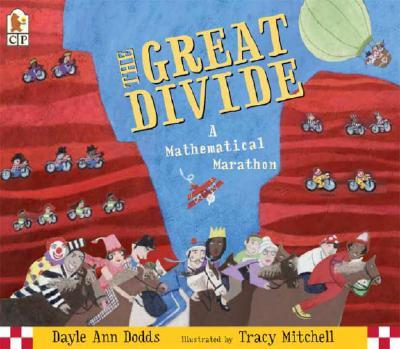## The Great Divide: A mathematical marathon

##### Australian Curriculum:  Description

This book contextualises division using a cycling marathon. Students can mentally calculate how many racers are left after each mishap then check as they turn the pages.

##### Teaching ideas
(1) Have students develop their own context/idea/story for division, have them see if their elbow partner can mentally calculate the correct answer; (2) Have students use numbers and symbols to represent the number sentences in the story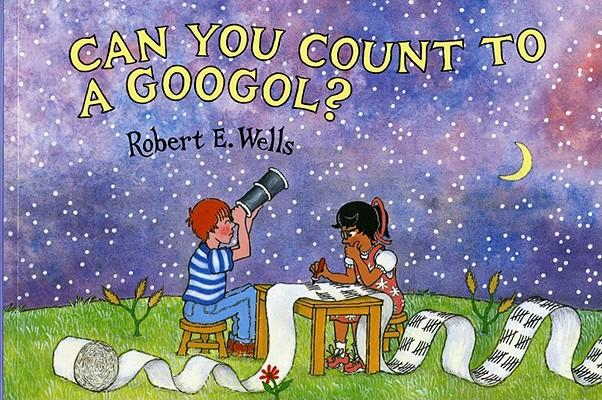## Can you count to a googol?

##### Australian Curriculum:  Description

Recognise, model, represent and order numbers to at least 10 000 (ACMNA052); Apply place value to partition, rearrange and regroup numbers to at least 10 000 to assist calculations and solve problems (ACMNA053); 4-Recognise, represent and order numbers to at least tens of thousands (ACMNA072); Apply place value to partition, rearrange and regroup numbers to at least tens of thousands to assist calculations and solve problems (ACMNA073); 7-Investigate index notation and represent whole numbers as products of powers of prime numbers (ACMNA149)

##### Teaching ideas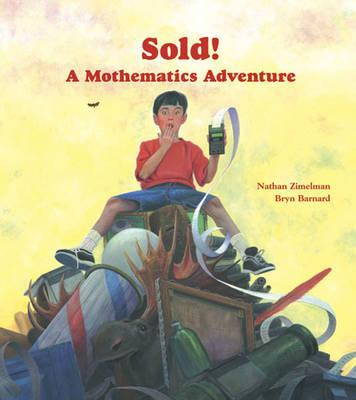##### Australian Curriculum:  Description

Create simple financial plans (ACMNA106)

##### Teaching ideas
Create basic financial plans.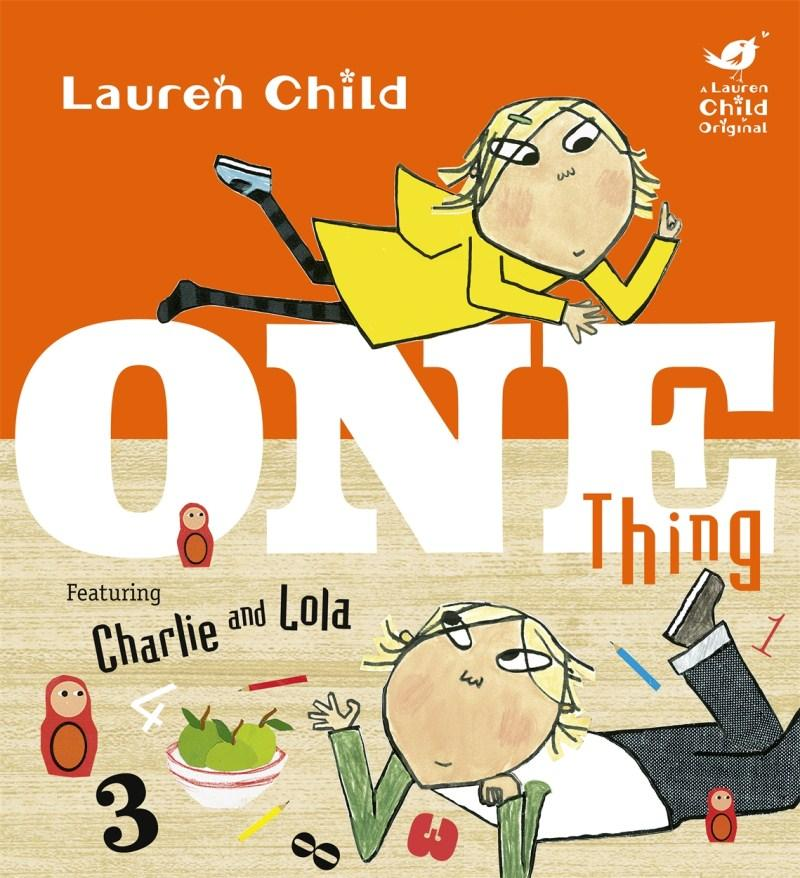## One Thing : featuring Charlie and Lola

##### Australian Curriculum:  Description

F.Y-Connect number names, numerals and quantities, including zero, initially up to 10 and then beyond (ACMNA002); Yr1-Represent and solve simple addition and subtraction problems using a range of strategies including counting on, partitioning and rearranging parts (ACMNA015); Yr2-Explore the connection between addition and subtraction (ACMNA029); Yr3-Recognise, model, represent and order numbers to at least 10 000 (ACMNA052); Yr3-Tell time to the minute and investigate the relationship between units of time (ACMMG062); Yr4-Recognise, represent and order numbers to at least tens of thousands (ACMNA072); Yr5-Convert between units of time (ACMMG085); Yr6-Select and apply efficient mental and written strategies and appropriate digital technologies to solve problems involving all four operations with whole numbers (ACMNA123)

##### Teaching ideas
Teach other vocabulary that is often used differently in everyday language such as the examples in the book; e.g. "how about no things" meaning 0 things. Explore time and how long the morning routine takes students in the class. Counting and counting on items.## Spaghetti and Meatballs for All

##### Australian Curriculum:  Description

Calculate perimeter and area of rectangles using familiar metric units (ACMMG109)

##### Teaching ideas
Pages at the back give teachers ideas and extension activities. E.g. How else could she have configured her dining room for the 32 guests? Cut and paste to assist students at the representational level of understanding. Create table arrangements for 12, 16, 24, 36 guests coming to the party.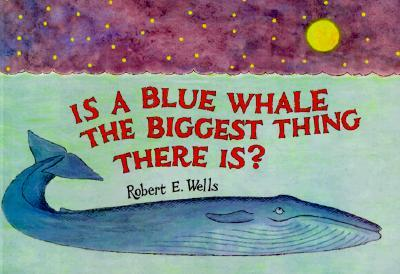## Is a Blue Whale the Biggest thing there is?

##### Australian Curriculum:  Description

Yr4 – Recognise, represent and order numbers to at least tens of thousands (ACMNA072); Yr5 – Choose appropriate units of measurement for length, area, volume, capacity and mass (ACMMG108);

##### Teaching ideas
Exploring some of these abstract concepts with concrete materials. Having the students create their own relationships between objects to aid in understanding large numbers, sizes and quantities.## Sir Cumference and the Sword in the Cone

##### Australian Curriculum:  Description

Year 5 – Measurement and Geometry : Shape – Connect three-dimensional objects with their nets and other two-dimensional representations (ACMMG111); Year 6 – Measurement and Geometry : Shape – Construct simple prisms and pyramids (ACMMG140); Year 7 – Measurement and Geometry : Shape – Draw different views of prisms and solids formed from combinations of prisms (ACMMG161); Year 8 – Measurement and Geometry : Shape – Investigate the relationship between features of circles such as circumference, area, radius and diameter. Use formulas to solve problems involving circumference and area (ACMMG197).

##### Teaching ideas
* Year 5 students could use page 5 to "form the solids and find their places"; * Year 6 students could use page 5 to "form the solids and find their places"; * Year 7 students could investigate Euler's Law by folding nets to form prisms and pyramids. They =could then draw up an investigation table (page 12) and draw their own conclusions; * By year 8 the mathematical vocabulary students know should enable them to understand and apprecaite the entire book. The teacher could pose the problem from the book without reading it, and have students work in groups to solve it. Were the steps you took to solving it the same as Radius and Vertex's?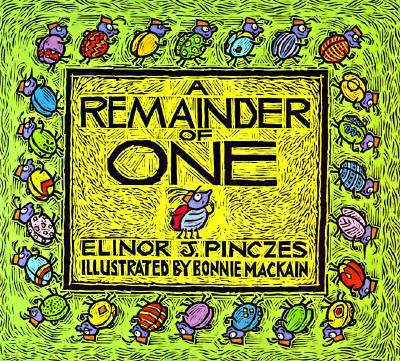## A Remainder of One

##### Australian Curriculum:  Description

Number and Algebra : Number and Place Value – Solve problems involving division by a one digit number, including those that result in a remainder (ACMNA101); Investigate index notation and represent whole numbers as products of powers of prime numbers (ACMNA149)

##### Teaching ideas
* Have students mathematically represent the ideas in the book. i.e. concrete with actual bugs, representational with tally marks and abstract would be the mathematical numbers and symbols (25 ÷ 2 = 12 r1; 25 ÷ 3 = 8 r1; 25 ÷ 4 = 6 r1; 25 ÷ 5 = 5). Year 7s could use this as an introduction to prime numbers and non-prime numbers. What are the factors of 24 and 25.## Divide & Ride

##### Australian Curriculum:  Description

Solve problems involving division by a one digit number, including those that result in a remainder (ACMNA101)

##### Teaching ideas
"Student investigate other ""Total Amounts"". How many people can go on the ride? What is the best number of people to go to the fair so that there is nobody sitting alone?"